Select Page
Tags: , , ,
0
(0)

The easiest way to communicate with Arduino is to use the serial port. It is also the most powerful tool to test and debug your code.

## Material

• Computer
• Arduino UNO x2
• Jumper cable M/M x3

## Communication with the Serial Monitor

The functions from the Serial library to know:

• Serial.begin() to initialize communication speed. Communnication will not work if this is not properly setup
• Serial.print() and Serial.println() to send strings. Last one send the command with an end-of-line ‘\n’
• Serial.write() to send one byte at a time
• Serial.available() to verify if data are present in the port’s buffer

Upload the following code to test communication between the computer and the Arduino.

```char cmd="";
char old_cmd;
boolean enable=false;

void setup(){
// Initialize serial port
Serial.begin(9600);
Serial.println("ENTER Commands:");
}

void loop(){
old_cmd=cmd;
if (Serial.available()){ // Verify that data are available
}

if(cmd!=old_cmd){
if(cmd=='O'){
Serial.println("Set to ON"); // Send data to serial port
enable=true;
}else if(cmd=='F'){
Serial.println("Set to OFF"); // Send data to serial port
enable=false;
}
}

if(enable){
Serial.println("System is running");
delay(200);
}
}

```

If you type O then F in the monitor command bar, you should see the following display:## Communication between two Arduino boards

It is possible to communicate between different devices using serial port such as two Arduino boards. One will be programmed as “Master” (give orders) the other one as “Slave” (receive order and react).

A serial port is defined by two wires and a communication speed. The SoftwareSerial.h library is used to defined serial ports. In this example, we choose pins 2 and 3 as reception (RX) and transmisson (TX) pin respectively (SoftwareSerial ArduinoSlave(2,3);). The communication speed rate is 9600bps (ArduinoSlave.begin(9600);).

To establish a correct communication, the boards should be wired correctly and the grounds linked as shown in the following schematics.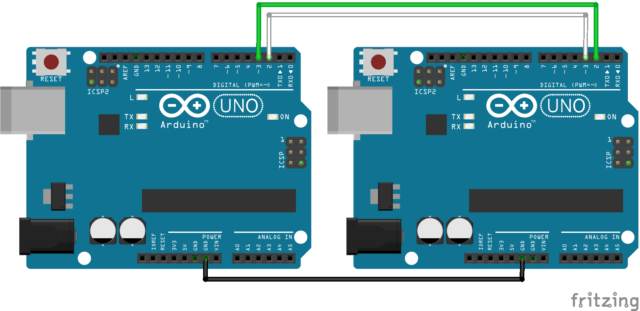In our example, the Master board send data and the Slave board receive and send it back.

### “Master” code

```#include <SoftwareSerial.h>

SoftwareSerial ArduinoSlave(2,3);
char cmd="";
char old_cmd;

void setup(){
Serial.begin(9600);
Serial.println("ENTER Commands:");
ArduinoSlave.begin(9600);
}

void loop(){
old_cmd=cmd;
if (Serial.available()){
}
if (ArduinoSlave.available()){
}
//Send data to slave
if(cmd!=old_cmd){
Serial.print("Master sent : ");
Serial.println(cmd);
ArduinoSlave.write(cmd);
}
}
}

```

### “Slave” code

```#include <SoftwareSerial.h>

SoftwareSerial ArduinoMaster(2,3);
char cmd="";
char old_cmd;

void setup(){
ArduinoMaster.begin(9600);
}

void loop(){
old_cmd=cmd;
if (ArduinoMaster.available()){
}
if(cmd!=old_cmd){
ArduinoMaster.write(cmd);
}
}

```

In the serial monitor of the Master board, if you type a command with one character, you should see the slave board answering back.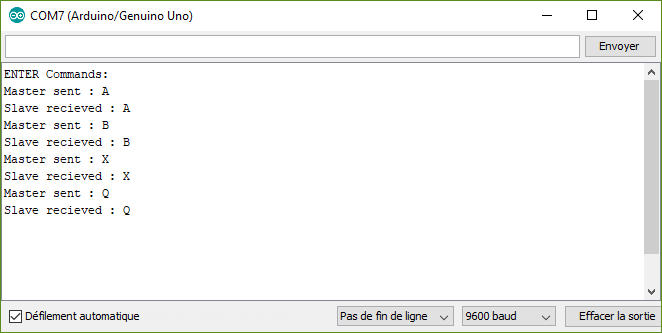With this code you can send and receive one byte at a time. The characters are coded following the ASCII table. Check the table ASCII to see how they are encoded. Some characters cannot be sent such as “°” or accents “é”.

## Play with data

For some project, you will need to play with more than one integer or one character and send several data with different type of value. A qucik fix is to convert the value into a string and send it with the print() function.

### Master code

```#include <SoftwareSerial.h>

SoftwareSerial ArduinoSlave(2,3);
String msg;

void setup(){

Serial.begin(9600);
Serial.println("ENTER Commands:");
ArduinoSlave.begin(9600);

}

void loop(){

//Send data to slave
if(msg!=""){
Serial.print("Master sent : ");
Serial.println(msg);
ArduinoSlave.print(msg);
msg="";
}

}

while (Serial.available()) {
delay(10);
if (Serial.available() >0) {
char c = Serial.read();  //gets one byte from serial buffer
msg += c; //add to String
}
}
Serial.flush(); //clean buffer
}

```

### Slave code

```#include <SoftwareSerial.h>

SoftwareSerial ArduinoMaster(2,3);
String msg;

void setup(){
Serial.begin(9600);
ArduinoMaster.begin(9600);
}

void loop(){

if(msg!=""){
ArduinoMaster.print(msg);
Serial.print("Master sent : " );
Serial.println(msg);
msg="";
}
}

while (ArduinoMaster.available()) {
delay(10);
if (ArduinoMaster.available() >0) {
char c = ArduinoMaster.read();  //gets one byte from serial buffer
msg += c; //makes the string readString
Serial.println(b);
}
}
ArduinoMaster.flush();
}

```

With this code you can send any type of data.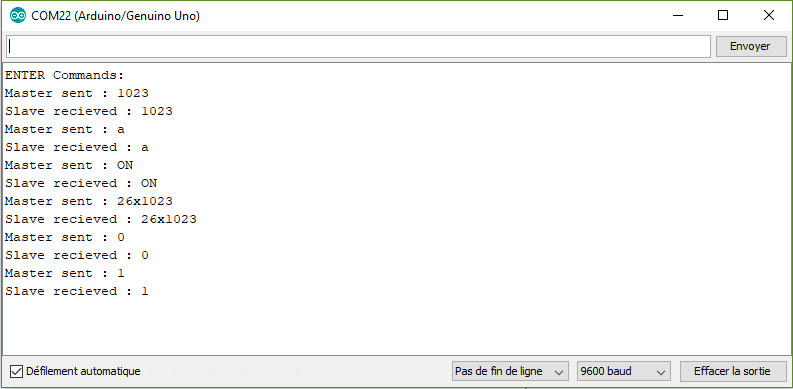Once the commmuniction is correctly setup and boards can exchange data, it is time to think about what to do with it.

## Send and receive sensor value

A good application is to change the light of a LED hooked up to the Slave board with a sensor on the Master board. We exchange Strings between boards that we must convert into integers. To Do so we use the funcntion atoi(). PWM value to control the LED is between 0 and 255 and the signal from the sensor is between 0 and 1023. We can use the map() function to convert value between those two ranges.

### Master code to read and send sensor value

```#include <SoftwareSerial.h>

SoftwareSerial ArduinoSlave(2,3);
String msg;
int intVal=0,oldIntVal=0;

void setup(){

Serial.begin(9600);
Serial.println("ENTER Commands:");
ArduinoSlave.begin(9600);

}

void loop(){

//Send data to slave
if(oldIntVal!=intVal){
Serial.print("Master sent : ");
Serial.println(intVal);
ArduinoSlave.print(intVal);
oldIntVal=intVal;
}
Serial.print("Slave LED PWM value : ");
}
delay(1000);
}

while (ArduinoSlave.available()) {
delay(10);
if (ArduinoSlave.available() >0) {
char c = ArduinoSlave.read();  //gets one byte from serial buffer
}
}
}
```

### Slave code to receive and use sensor value

```#include <SoftwareSerial.h>

SoftwareSerial ArduinoMaster(2,3);

#define ledPin 11

String msg="";
int ledVal=0;
int intVal=0,oldIntVal=0;

void setup(){
Serial.begin(9600);
ArduinoMaster.begin(9600);
pinMode(ledPin,OUTPUT);
}

void loop(){
convertMsgToCmd();
if(intVal!=oldIntVal){
Serial.print("Master sent : " );
Serial.println(intVal);

ledVal=map(intVal,0,1023,0,255);
Serial.print("led value : ");
Serial.println(ledVal);
ArduinoMaster.print(ledVal);
analogWrite(ledPin,ledVal);
oldIntVal=intVal;
}
}

while (ArduinoMaster.available()) {
delay(10);
if (ArduinoMaster.available() >0) {
char c = ArduinoMaster.read();  //gets one byte from serial buffer

msg += c; //makes the string readString
}
}
ArduinoMaster.flush();
}

void convertMsgToCmd(){
if (msg.length() >0) {
Serial.print("message length : ");
Serial.println(msg.length());

char carray1; //magic needed to convert string to a number
msg.toCharArray(carray1, sizeof(carray1));
intVal = atoi(carray1);

msg="";
}
}

```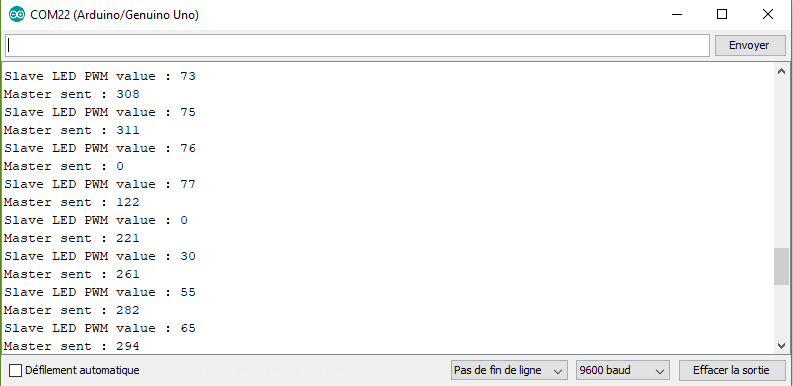## Send and receive two sensor values

To recieve several values, the idea is to define a separator. In our case, we select the letter “x”. We just need to find the place of the character in the String with the function indexOf().

### Master code to read and send two sensor values

```#include <SoftwareSerial.h>

SoftwareSerial ArduinoSlave(2,3);
String msg;
int intVal1=0,oldIntVal1=0;
int intVal2=0,oldIntVal2=0;

void setup(){
Serial.begin(9600);
Serial.println("ENTER Commands:");
ArduinoSlave.begin(9600);

}

void loop(){

//Send data to slave
if(oldIntVal1!=intVal1 || oldIntVal2!=intVal2 ){
Serial.print("Master sent : ");
Serial.print(intVal1);
Serial.print("x");
Serial.println(intVal2);

ArduinoSlave.print(intVal1);
ArduinoSlave.print("x");
ArduinoSlave.print(intVal2);
oldIntVal1=intVal1;
oldIntVal2=intVal2;
}
delay(1000);

}

}

while (Serial.available()) {
delay(10);
if (Serial.available() >0) {
char c = Serial.read();  //gets one byte from serial buffer
msg += c; //makes the string readString
}
}
Serial.flush();
}

while (ArduinoSlave.available()) {
delay(10);
if (ArduinoSlave.available() >0) {
char c = ArduinoSlave.read();  //gets one byte from serial buffer
}
}
}

```

### Slave code to receive two sensor values

```#include <SoftwareSerial.h>

SoftwareSerial ArduinoMaster(2,3);

String msg="",m1="",m2="";
int num1=-1,num2=-1;
int sep;

void setup(){
Serial.begin(9600);
ArduinoMaster.begin(9600);
}

void loop(){
convertMsgToMultiCmd();
if(num1!=-1 && num2!=-1){
Serial.print("Sensor 1 : " );
Serial.println(num1);

Serial.print("Sensor 2 : " );
Serial.println(num2);

ArduinoMaster.print("Sensor 1 : " );
ArduinoMaster.println(num1);

ArduinoMaster.print("Sensor 2 : " );
ArduinoMaster.println(num2);
num1=-1;
num2=-1;
}
}

while (ArduinoMaster.available()) {
delay(10);
if (ArduinoMaster.available() >0) {
char c = ArduinoMaster.read();  //gets one byte from serial buffer

msg += c; //makes the string readString
}
}
ArduinoMaster.flush();
}

void convertMsgToMultiCmd(){
if (msg.length() >0) {
Serial.print("message length : ");
Serial.println(msg.length());
sep = msg.indexOf('x');
//Serial.println(sep);
m1 = msg.substring(0, sep); //get servo id
m2 = msg.substring(sep+1, msg.length()); //get servo pos

char carray1; //magic needed to convert string to a number
m1.toCharArray(carray1, sizeof(carray1));
num1 = atoi(carray1);

char carray2;
m2.toCharArray(carray2, sizeof(carray2));
num2 = atoi(carray2);

msg="";
}
}

```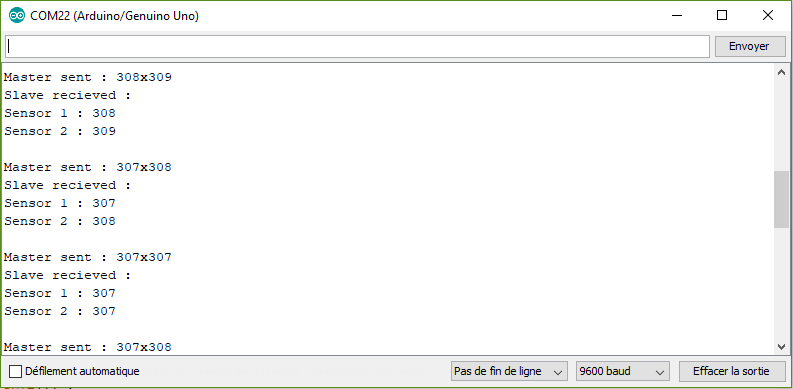## Send and receive multiple data

It is possible in your application, that you need to exchange several data. Inthis case, we need to change the conversion function. In this example, we want to control a led state, the speed and direction of motor.

### Master code to send multiple data

```#include <SoftwareSerial.h>

SoftwareSerial ArduinoSlave(2,3);
String msg;
int intVal1=0,oldIntVal1=0;
int intVal2=0,oldIntVal2=0;

void setup(){

Serial.begin(9600);
Serial.println("ENTER Commands:");
ArduinoSlave.begin(9600);

}

void loop(){

//Send data to slave
if(msg!=""){
Serial.print("Master sent : ");
Serial.println(msg);
ArduinoSlave.print(msg);
msg="";
}

}
delay(1000);
}

while (Serial.available()) {
delay(10);
if (Serial.available() >0) {
char c = Serial.read();  //gets one byte from serial buffer
msg += c; //makes the string readString
}
}
Serial.flush();
}

while (ArduinoSlave.available()) {
delay(10);
if (ArduinoSlave.available() >0) {
char c = ArduinoSlave.read();  //gets one byte from serial buffer
}
}
}

```

### Slave code to receive and use multiple data

```#include <SoftwareSerial.h>
SoftwareSerial ArduinoMaster(2,3);
String msg="";
int ledVal=0;
int sep;
String data;
unsigned int data_count=0;
void setup(){
Serial.begin(9600);
ArduinoMaster.begin(9600);
}
void loop(){
convertMsgToMultiCmd();
//Use data
if(data_count==3){
for(int i=0;i<(data_count+1);i++){
switch(i){
case 0: //led status
if(data=="ON"){
Serial.println("Switch led ON");
}else if(data=="OFF"){
Serial.println("Switch led OFF");
}else{
Serial.println("Led wrong command");
}
break;
case 1: //motor PWM
Serial.print("Set motor power to : ");
Serial.println(stringToInt(data));
break;
case 2: //motor direction
if(data=="forward"){
Serial.println("Motor direction forward");
}else if(data=="backward"){
Serial.println("Motor direction backward");
}else{
Serial.println("Motor wrong command");
}
break;
}
}
data_count=0;
}
}
while (ArduinoMaster.available()) {
delay(10);
if (ArduinoMaster.available() >0) {
char c = ArduinoMaster.read();  //gets one byte from serial buffer
msg += c; //makes the string readString
}
}
ArduinoMaster.flush();
}
void convertMsgToMultiCmd(){
if (msg.length() >0) {
data_count=0;
Serial.print("Master sent : ");
Serial.println(msg);//Serial.println(msg.length());
do{
sep = msg.indexOf('x');
// expect a string like 0x0021 containing the two servo positions
String m1 = msg.substring(0, sep); //get servo id
msg = msg.substring(sep+1, msg.length()); //get servo pos
data[data_count]=m1;
data_count++;
} while(sep!=-1);
for(int i=0;i<(data_count+1);i++) Serial.println(data[i]);
msg="";
}
}
int stringToInt(String s){
char carray1; //magic needed to convert string to a number
s.toCharArray(carray1, sizeof(carray1));
return atoi(carray1);
}
```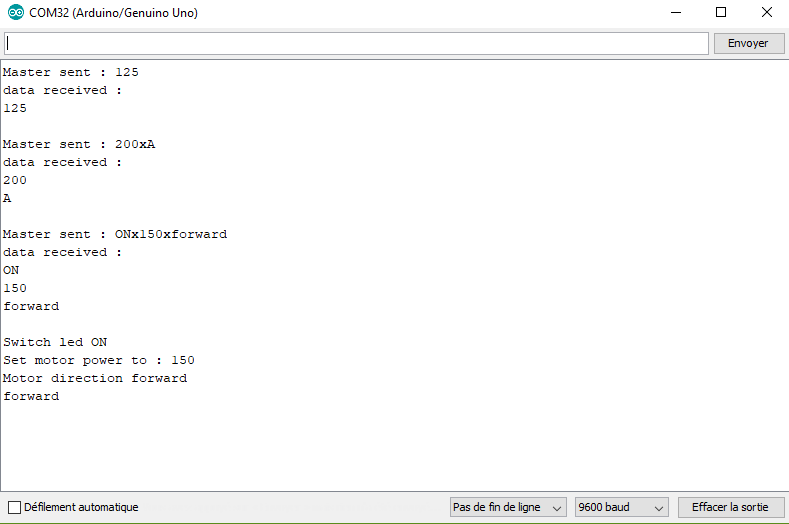Once you can receive and separate the data, you can use them as you like. Convert into integer sensor value or use specific String to trigger actions.

With this basic knowledge, you should be able to communicate between devices such as serial servo controller, Bluetooth module or other microcontroller such as Raspberry Pi.

If you’re having a hard time establishing a communication between two devices or if you’d like other example, leave a comment or send us a message.

## Sources

Find other examples and tutorials in our Automatic code generator

Click on a star to rate it!

Average rating 0 / 5. Vote count: 0

No votes so far! Be the first to rate this post.

As you found this post useful...